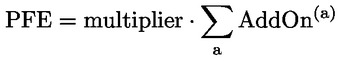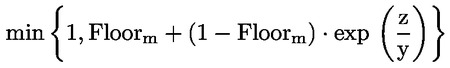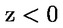In force

Version from: 01/01/2023
Amendments
Search within this legal act

### Article 278 - Potential future exposure

Article 278

Potential future exposure

1.

Institutions shall calculate the potential future exposure of a netting set as follows:where:

 PFE = the potential future exposure;
 a = the index that denotes the risk categories included in the calculation of the potential future exposure of the netting set;
 AddOn(a) = the add-on for risk category a calculated in accordance with Articles 280a to 280f, as applicable; and
 multiplier = the multiplication factor calculated in accordance with the formula referred to in paragraph 3.

For the purpose of this calculation, institutions shall include the add-on of a given risk category in the calculation of the potential future exposure of a netting set where at least one transaction of the netting set has been mapped to that risk category.

2.
The potential future exposure of multiple netting sets that are subject to one margin agreement, as referred in Article 275(3), shall be calculated as the sum of the potential future exposures of all the individual netting sets as if they were not subject to any form of a margin agreement.
3.

For the purposes of paragraph 1, the multiplier shall be calculated as follows:

multiplier =1 if z ≥ 0ifwhere:

Floorm = 5 %;
y = 2 · (1 – Floorm) · ΣaAddOn(a)

z =CMVNICA for the netting sets referred to in Article 275(1)

CMV – VM – NICA for the netting sets referred to in Article 275(2)

CMViNICAi for the netting sets referred to in Article 275(3)

 NICAi = the net independent collateral amount calculated only for transactions that are included in netting set i. NICAi shall be calculated at trade level or at netting set level depending on the margin agreement.